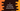# C program to compare two strings using strcmp# Introduction :

In this tutorial, we will learn how to compare two strings in C using strcmp() method. This method takes two arguments i.e. the two strings and returns one integer value. It is defined as below :

## Definition of strcmp :

The strcmp method is defined as below :

``````int strcmp(const char *s1, const char *s2)
``````

## Parameter and Return value :

Following are the meaning of its parameters :

s1 : The first string s2 : The second string

## Return value :

It returns one integer value. It can be equal to 0, less than 0 and greater than 0.

• If it is equal to 0, it means that both strings are equal.
• If it is greater than 0, the first string is greater than the second string.
• If it is less than 0, the first string is smaller than the second string.

### Example program :

Let me show you one example how it works :

``````#include <stdio.h>
#include <string.h>

void compareStrings(char *a, char *b)
{
int result = strcmp(a, b);
if (result > 0)
{
printf("'%s' is greater than '%s'\n", a, b);
}
else if (result < 0)
{
printf("'%s' is less than '%s'\n", a, b);
}
else
{
printf("'%s' is equal to '%s'\n", a, b);
}
}
int main()
{
char firstStr[] = "Hello";
char secondStr[] = "World";
char thirdStr[] = "Hello";

compareStrings(firstStr, secondStr);
compareStrings(firstStr, thirdStr);
}``````

### Explanation :

1. We are including the string.h header at the start of the program. strcmp is defined in this header.
2. We have created one function to do the comparison : compareStrings. This function takes two char * arguments and prints out the result.
3. compareStrings compares two strings using the strcmp function. It uses one if-else if-else block to print the final result.
4. We are calling this function from the main() function.

### Output :

The output of the above program is as below :

``````'Hello' is less than 'World'
'Hello' is equal to 'Hello'
``````

### Conclusion :

In this tutorial, we have learned how to compare two strings using strcmp method. We have used one separate function to write the string comparison logic. Using a separate function is always helpful if you want to use a piece of code multiple times. Try to go through the example and drop one comment below if you have any queries.